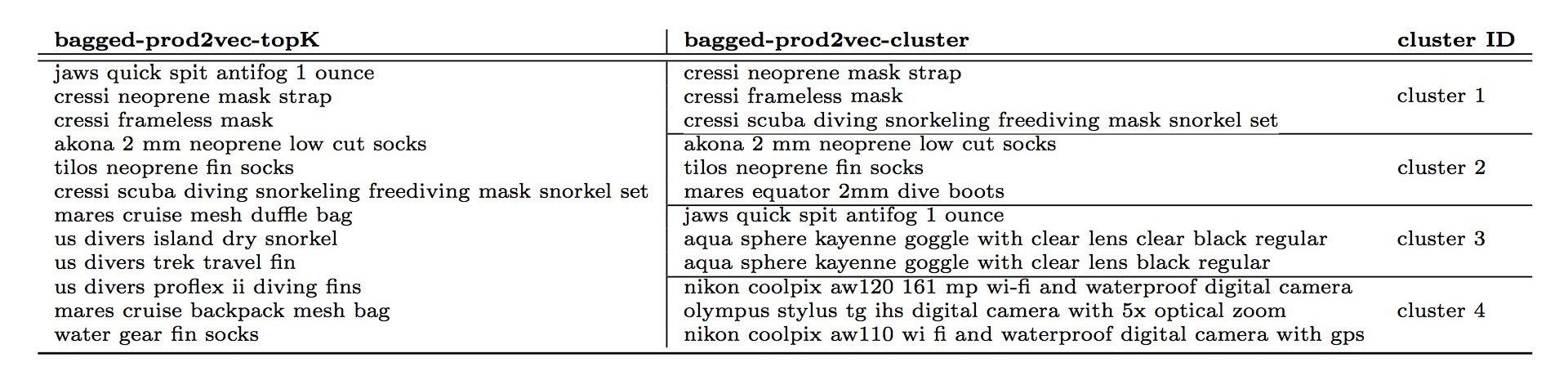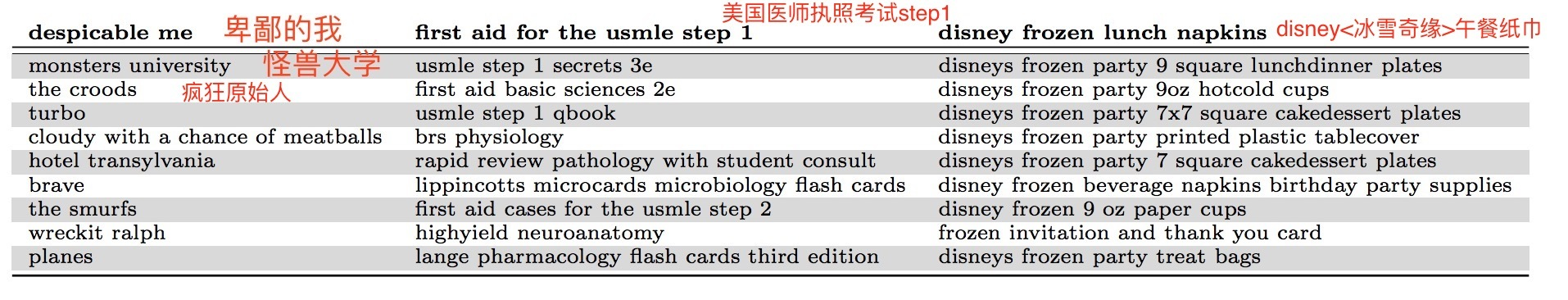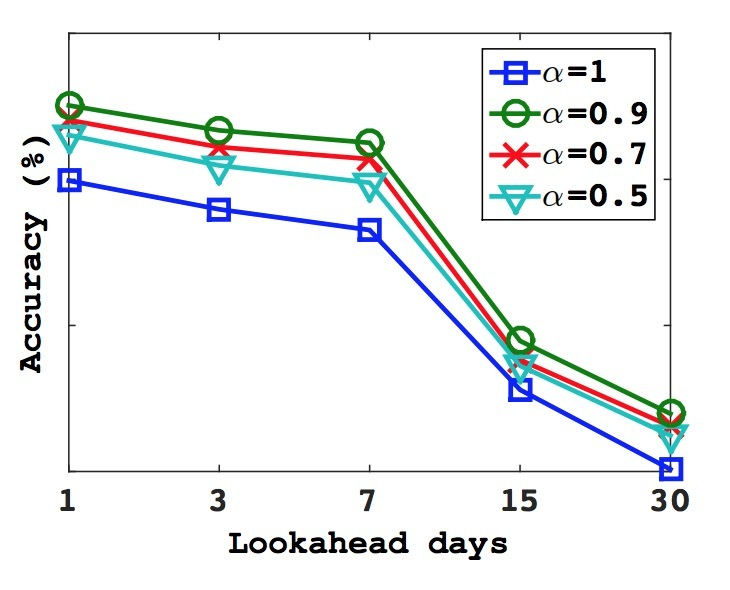# 介绍# 2.系统总览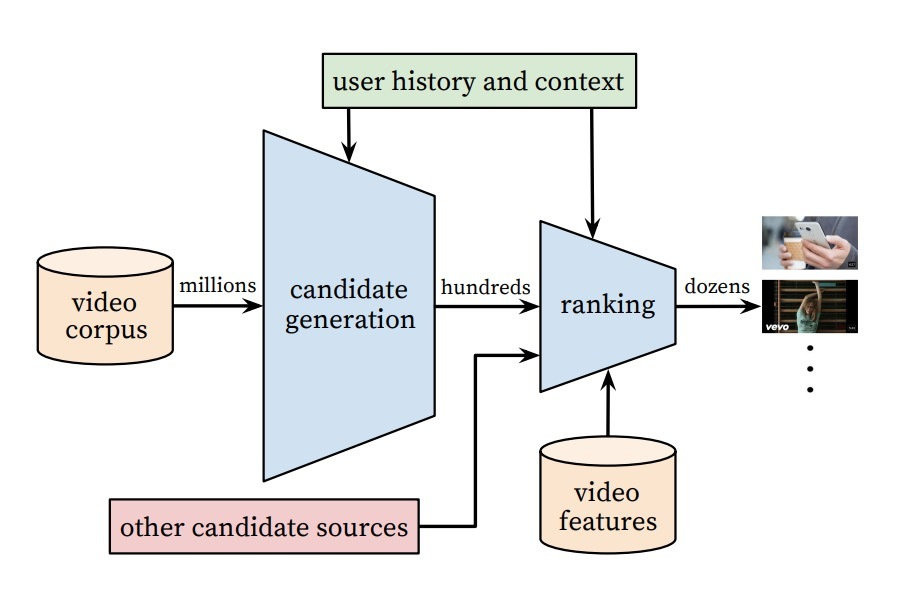# 一、候选生成

## 3.1 推荐即分类

Efficient Extreme Multiclass

## 1.1 模型架构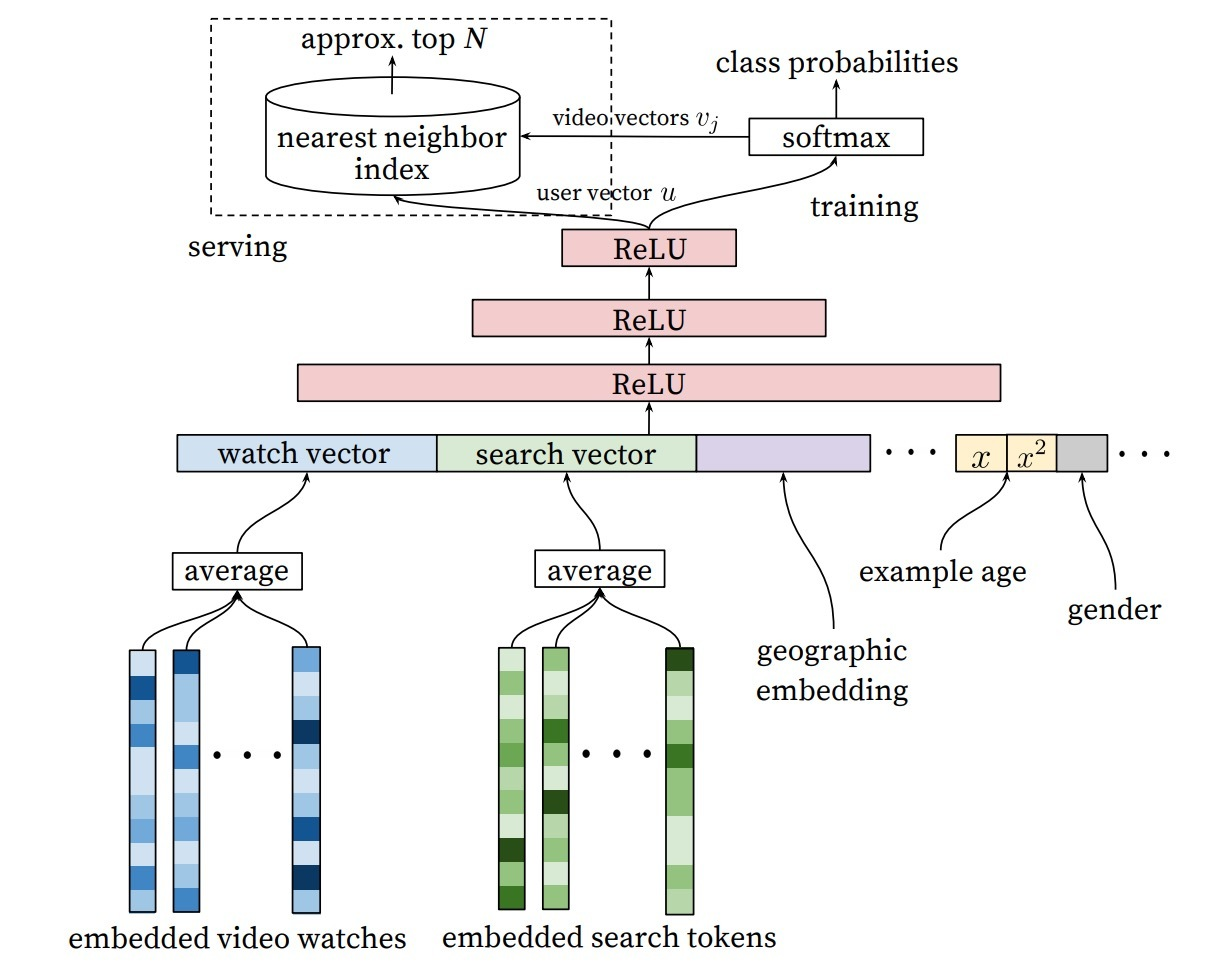## 1.2 多种信号

“样本时限”特征（”Example Age” Feature）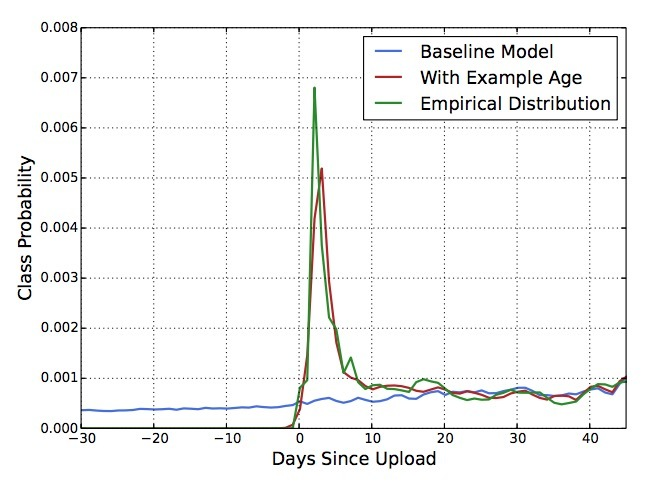## 1.3 Label和Context选择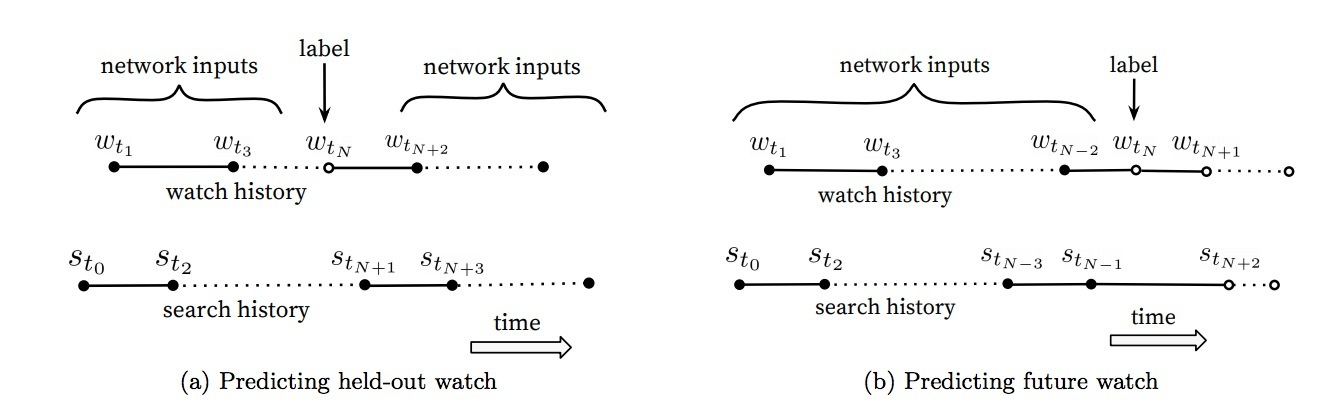## 1.4 特征和深度的试验

• Depth 0: 一个线性层，可简单地将串联层转换成与softmax相匹配的256维.
• Depth 1: 256 ReLU
• Depth 2: 512 ReLU -> 256 ReLU
• Depth 3: 1024 ReLU -> 512 ReLU -> 256 ReLU
• Depth 4: 2048 ReLU -> 1024 ReLU -> 512 ReLU -> 256 ReLU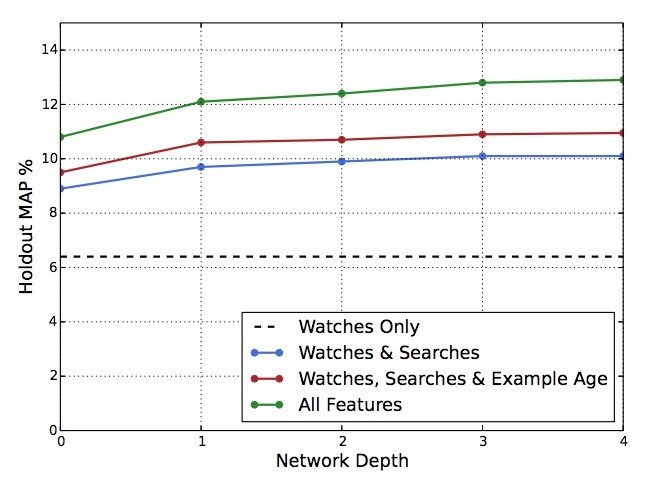# 二、Ranking

Ranking的主要作用是，针对指定的UI，使用曝光数据来特化和校正候选预测（specialized and calibrate candidate predictions）。例如，用户通常会观看一个probability值较高的视频，但不大可能去点击指定主页上缩略图的曝光。在Ranking时，我们会访问许多描述视频的特征、以及视频与用户关系的特征，因为在候选集生成阶段，只有一小部分的视频被打过分，而非上百w的视频。Ranking对于聚合不同的候选源很重要，因为每个源的得分不能直接对比。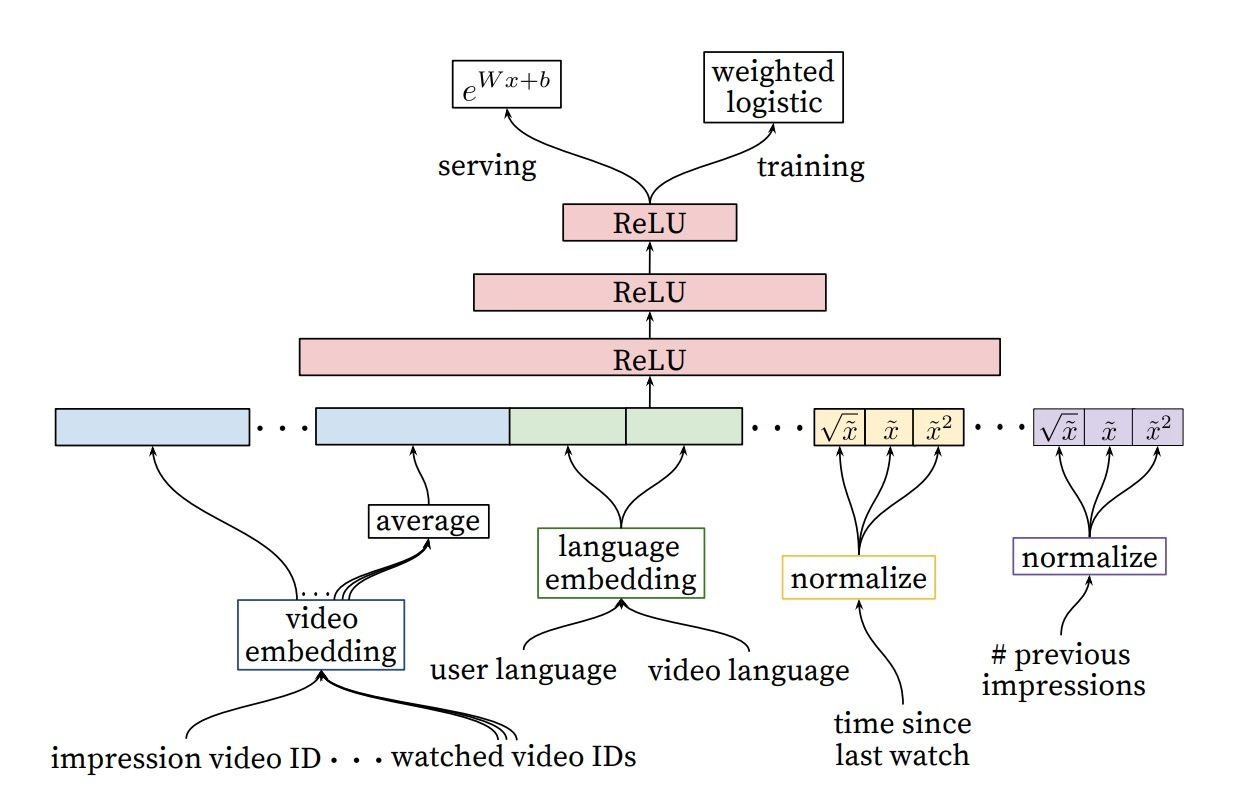## 2.3 隐层的试验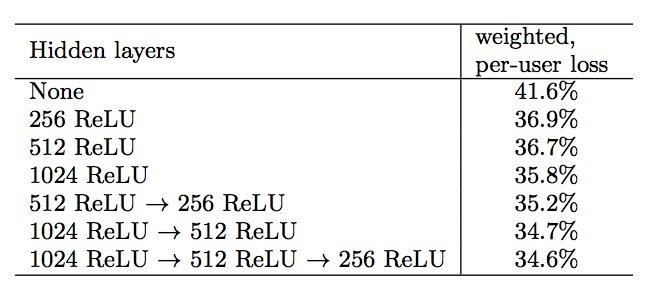# 3.层次化语言模型

## 3.1 模型结构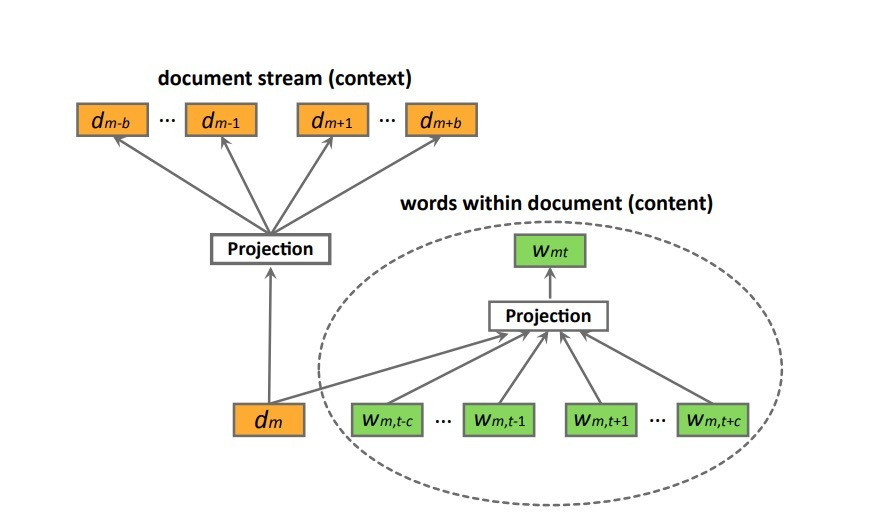$L = \sum_{s \in S} (\alpha \sum_{d_m \in s} \sum_{w_{mt} \in d_m} log P(w_{mt} | w_{m,t-c}: w_{m,t+c}, d) + \\ \sum _{d_m \in s} (\alpha log P(d_m | w_{m1}: w_{mT}) + \sum_{-b \le i \le b, i \neq 0} log P(d_{m+i} | d_m)))$ …(8)

$P(d_{m+i} | d_{m}) = \frac{exp(v_{d_m}^T v_{d_{m+i}}')} {\sum_{d=1}^{M} exp(v_{d_m}^T v_d')}$ …(9)

$P(w_{mt} | w_{m,t-c}: w_{m,t+c}, d_m) = \frac{exp(\bar{v}^T v_{w_{mt}}')} {\sum_{w=1}^{W} exp(\bar{v}^T v_w')}$ …(10)

$\bar{v} = \frac{1}{2c+1} (v_{d_m} + \sum_{-c \leq j \leq c, j \neq 0} v_{w_{m,t+j}})$ …(11)

## 3.3 模型最优化

$P(d_{m+i} \mid d_m) = \prod_l P(h_l \mid q_l, d_m)$ …(12)

$P(h_l = 1 \mid q_l, d_m) = \sigma(v_{d_m}^T V_{q_l})$ …(13)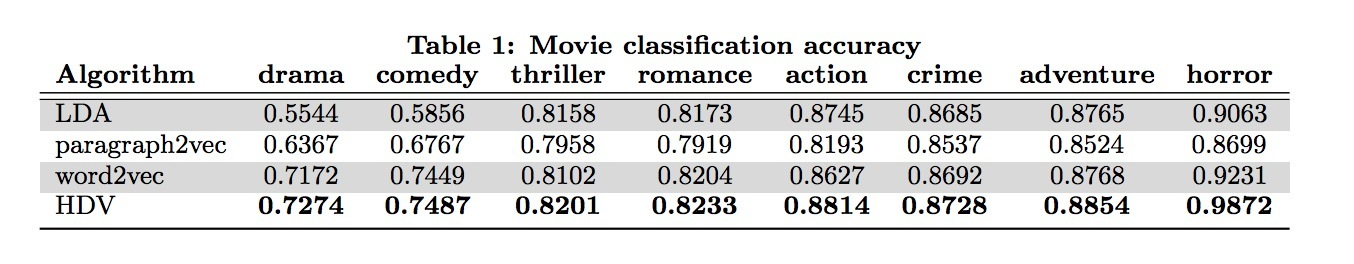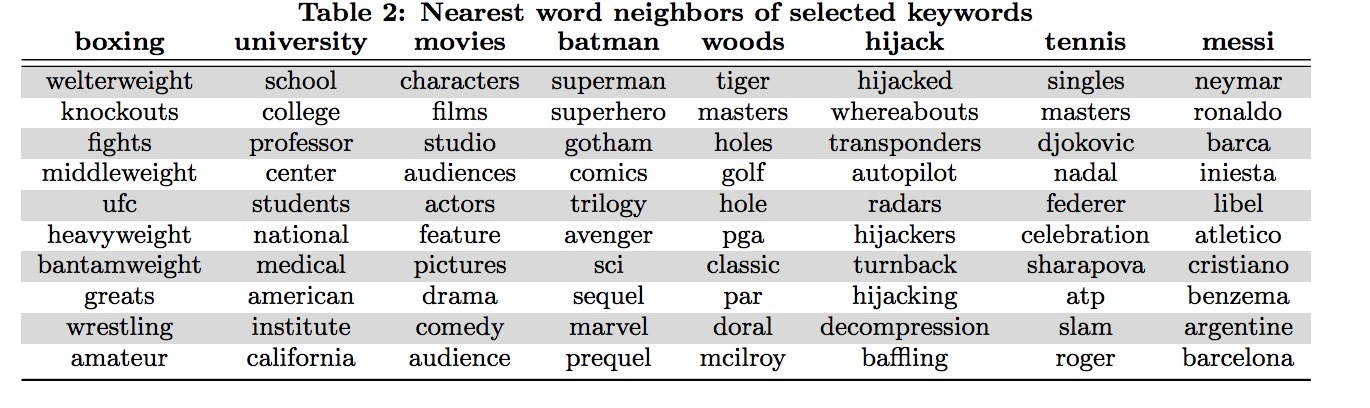# 1.介绍DeepWalk会将一个图作为输入，并生成一个隐表示作为它的输出。将我们的方法应用于Karate network上，结果如图1所示。图1a通过力导向（force-directed）的布局进行表示，图1b展示了我们使用2个隐维度的方法。除了显著的相似性外，我们注意到1b中线性可分的部分，对应于通过输入图1a的模块最大化（modularity maximization）的聚类（clusters）: 通过顶点颜色区分。

DeepWalk比其它用于创建社交维度（social dimension）隐表示方法要好，尤其是当带label的节点（labeled nodes）很稀少时。我们的表示法的性能可能与最简单的分类器（LR）相当。我们的表示是可泛化的，可以结合任何分类方法（包含迭代型推断法）。DeepWalk可以完成上述所有，它是一个在线算法，可并行化。

• 引入深度学习作为一个工具来分析图，来构建健壮的表示，很适合于统计建模。DeepWalk会学到在短随机游走(short random walks)内表示的结构化规律。
• 我们对多个社交网络上使用我们的表示法，并在多标签分类上进行评估。我们发现可以极大提升存在标签稀疏性情况的分类效果，在Micro F1上可以提升5%-10%。在一些case中，即使只给了更少的60%的数据，DeepWalk的表示也可以胜出其它对手。

# 3.学习社交表示

• 社群意识（Community aware）：隐维度间的距离可以作为评估网络成员的社交相似性的一个metric。这允许泛化到同质的网络中。
• 低维（Low dimensional）：当标记数据很少时，低维模型泛化更好，加速收敛和推断。
• 连续（Continuous）：我们需要隐表示来建模在连续空间中的部分社群成员关系（community membership）。除了提供一个关于社群关系的细微视图外，连续表示法具有在社群间的平滑决策边界，这可以允许更健壮的分类。

## 3.1 随机游走

• 1.局部探索（local exploration）很容易并行化。多个随机游走者（random walkers）以不同线程、进程、机器可以并行探索同一个graph下的不同部分。
• 2.依靠从短随机游走上获取信息，可以使它更能适应在图结构上的小变动，无需进行全局重新计算。我们可以迭代式地更新学到的模型，从变动区域上使用新的随机游走，它对整个graph是在时间上是次线性的。

## 3.2 连接：二八法则（power law）## 3.3 语言建模

$Pr(v_i | (\Phi(v_1), \Phi(v_2), ..., \Phi(v_{i-1})))$ …(1)

$minimize_{\Phi} - log Pr({v_{i-w}, ..., v_{i-1}, v_{i+1}, ..., v_{i+w}} | \Phi(v_i))$ …(2)

# 4.方法

## 4.2 算法：DeepWalk

• 1.随机游走生成器
• 2.一个更新过程### 4.2.1 SkipGram

SkipGram是一个语言模型，在一个句子中，它会最大化在同一窗口w中词之间的共现率。## 4.3 并行化(Parallelizability)# 参考

DeepWalk: Online Learning of Social Representations

# 1.介绍# 2.方法

## 2.1 引入skip-ghought vectors

Encoder：假设$w_i^1, ..., w_i^N$是句子$s_i$中的words，其中N表示在句子中的words数目。在每个step中，encoder会产生一个hidden state：$h_i^t$，它可以解释成序列$w_i^1,...,w_i^t$的表示（representation）。hidden state：$h_i^N$可以表示整个句子。

$r^t = \sigma(W_r x^t + U_r h^{t-1})$ … (1)

$z^t = \sigma(W_z x^t + U_z h^{t-1})$ … (2)

$\bar{h}^t = tanh(W x^t + U (r^t \odot h^{t-1})$ … (3)

$h^t = (1-z^t) \odot h^{t-1} + z^t \odot \bar{h}^t$ …(4)

Decoder: decoder是一个神经语言模型，它的条件是encoder output $h_i$。该计算与encoder相似，除了我们引入了矩阵$C_z，C_r$，以及C，它们被用于偏置由句子向量计算得到的update gate，reset gate和hidden state。一个decoder会被用于下一个句子$s_{i+1}$，而第二个decoder被用于前一句子$s_{i-1}$。Separate参数被用于每个decoder，除了词汇矩阵V，它的权重矩阵会连接decoder的hidden state，以便计算在词上的一个分布。我们在下一个句子$s_{i+1}$上描述decoder，通过一个在前一句子$s_{i-1}$上的类似计算得到。假设$h_{i+1}^t$表示decoder在时间t的hidden state。对下面的等式进行迭代（丢掉下标i+1）：

$r^t = \sigma(W_r^d x^{t-1} + U_r^d h^{t-1} + C_r h_i)$ …(5)

$z^t = \sigma(W_z^d x^{t-1} + U_z^d h^{t-1} + C_z h_i)$ …(6)

$\bar{h}^t = tanh(W^d x^{t-1} + U^d (r^t \odot h^{t-1} + C h_i$ …(7)

$h_{i+1}^t = (1-z^t) \odot h^{t-1} + z^t \odot \bar{h}^t$ …(8)

$% $ …(9)

$% $ …(10)

# 3 实验

• 使用学到的encoder作为一个feature extractor，抽取所有句子的skip-thought vectors
• 如果该任务涉及到计算句子对（pairs of sentences）之间的分数，会计算pairs间的component-wise features。
• 在抽取的features上训练一个线性分类器，在skip-thoughts模型中没有任何额外的fine-tuning或者backpropagation。

t-sne.

# 参考

Convolutional Neural Networks for Sentence Classification

# 1.介绍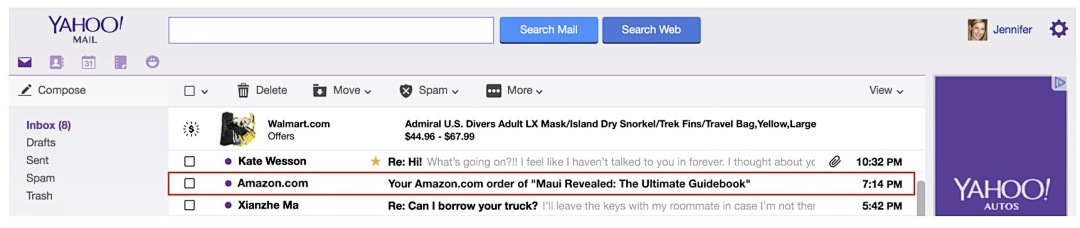# 3.方法

## 3.1 低维商品embeddings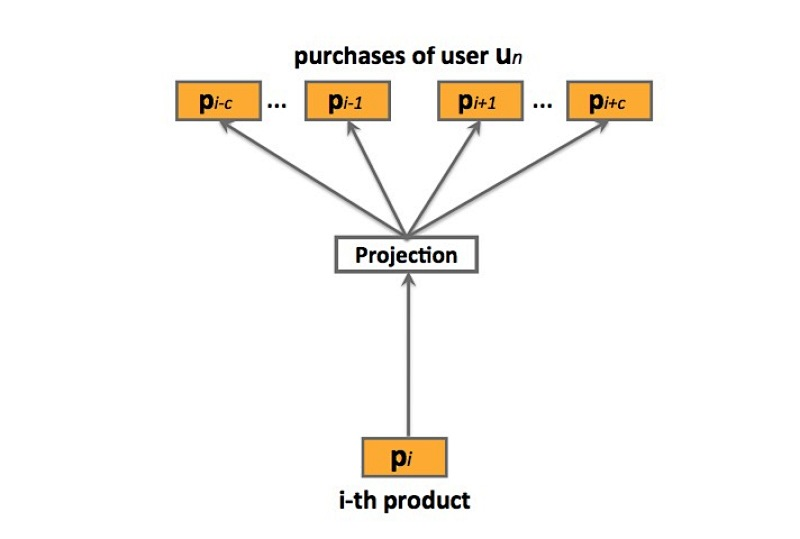prod2vec：prod2vec模型会将一个购买序列看成是一个“句子”，在这个序列中的商品看成是“词”。详见图2, 更特殊的，prod2vec使用skip-gram模型来学习商品表示，通过以下的最大化目标函数：

…(3.1)

…(3.2)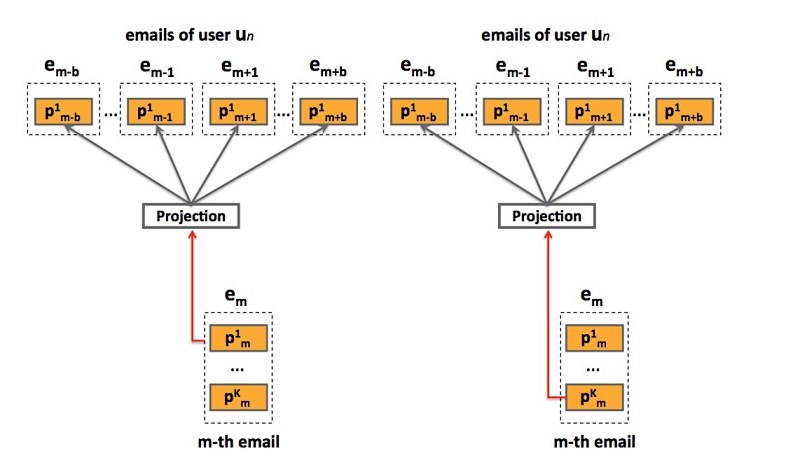bagged-prod2vec：为了对多个商品同时购买的行为做出解释，我们提出了一个修改版本的skip-gram模型，它引入了一个概念：购物袋（shopping bag）。如图3所示，该模型会在email receipts级别进行操作，而非在商品级别。通过对email序列s上的一个修改版目标函数进行最大化，来学习商品向量表示：

…(3.3)

learning：该模型使用SGA（ stochastic gradient ascent）进行最优化，很适合大规模问题。然而，在(3.1)和(3.3)中的梯度计算$\Delta L$，很适合词汇size P，实际任务中，计算开销会随着P的增大而变得很昂贵，很容易达到上百万的商品。另一方面，我们使用negative sampling方法，它能显著减小计算复杂度。

## 3.2 prod-2-prod预测模型

prod2vec-topK：给定一个购买商品，该方法会为所有在词汇表中的商品计算cosine相似度，并推荐最相似商品的top K

prod2vec-cluster：为了能做出更多样化(diverse)的推荐，我们考虑将相似商品分组成聚类，从与之前购买商品相关的聚类中推荐商品。我们应用K-means聚类算法来在hadoop FS上实现，将商品基于cosine相似度进行聚类。我们假设：在从聚类$c_i$上进行一个购买后、再从任意第C个聚类中进行一次购买的行为，符合一个多项式分布（multinomial distribution）$M_u(\theta_{i1}, \theta_{i2}, ..., \theta_{iC})$，其中$\theta_{ij}$是从聚类$c_i$中进行一次购买后、接着从聚类$c_j$中进行一次购买的概率。为了估计参数$\theta_{ij}$，对于每个i和j，我们采用一个最大似然方法：

$\hat {\theta_{ij}} = \frac{c_i购买后跟c_j的次数}{c_i购买的总数}$ …(3.4)

• count of ci purchases: c_i购买的数目
• # of times ci purchase was followed by cj: c_i购买后跟c_j的次数

## 3.3 User-to-product预测模型

user2vec：受paragraph2vec算法的启发，user2vec模型会同时学习商品和用户的特征表示，它会将用户当成是一个“全局上下文”。这样的模型如图4所示。训练数据集来自于用户购买序列S，它会包含$u_n$和其它已购商品（通过购买时间序排列），$u_n = (p_{n1}, p_{n2}, ..., p_{nU_n})$，其中$U_n$表示用户$u_n$购买的items数目。在训练期间，用户向量会被更新，来预测从他的email receipts中的商品，其中，学到的product vectors会预测在上下文中的其它商品。出于表示的简洁性，在下面，我们会表示no-bagged版本的模型，注意，使用bagged版本进行扩展也很方便。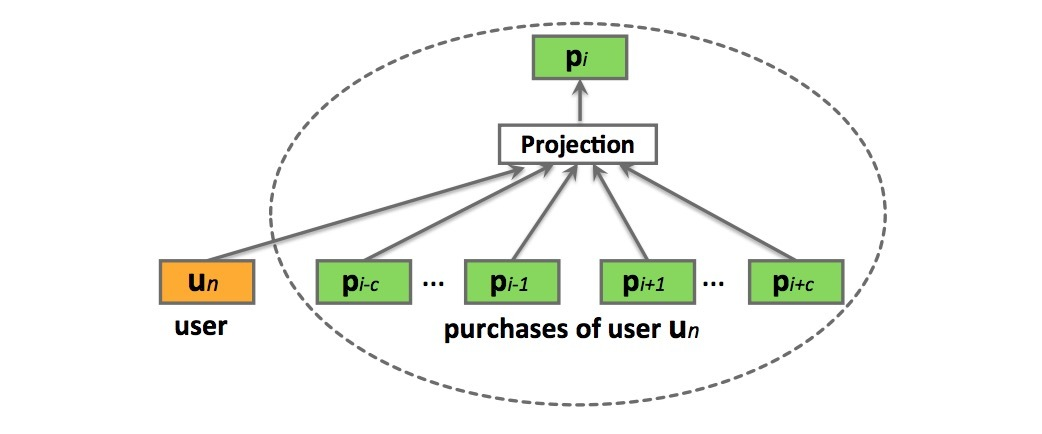…(3.5)

$P(p_{ni}|p_{n,i-c}: p_{n,i+c}, u_n) = \frac{e^{\bar{v}^T v_{p_{ni}}'}}{ \sum_{p=1}^{V} e^{\bar{v}^T v_p'}}$ …(3.6)

$\bar{v} = \frac{1}{2c+1} (v_{u_n} + \sum_{-c \leq j \leq c ,j \neq 0} v_{p_{n,i+j}})$ …(3.7)

$P(u_n | p_{n1}: p_{nU_n}) = \frac{e^{\bar{v}_n^T v_{u_n}'}} {\sum_{p=1}^V e^{\bar{v}_n^T v_p'}}$ …(3.8)

…(3.9)

user2vec模型的一个主要优点是，商品推荐是基于该用户的购买历史进行量身定制的。然而，缺点是，该需要需要非常频繁地进行更新，不同于product-to-product方法，它可以长期是相关的，而user-to-product推荐需要经常变化来对最近购买行为做出解释。

# 4.实验及其它

## 4.4 推荐预测商品

• 1) prod2vec-topK：使用数据集$D_p$进行训练，其中，商品向量通过对购买序列s通过极大似然估计进行学习。给定一个商品$p_i$，通过向量空间计算cosine相似度选择topK个相似商品。
• 2) bagged-prod2vec-topK：使用$D_p$进行训练，其中商品向量通过email序列s通过极大似然估计进行学习。对于给定商品$p_i$，通过选择在结合向量空间计算cosine相似度选择topK个相似商品。
• 3) bagged-prod2vec-cluster: 与bagged-prod2vec模型的训练类似，接着将商品向量聚类成C个聚类，并计算它们间的转移概率。接着标识出$p_i$属于哪个聚类（例如：$p_i \in c_i$），我们根据$c_i$的转移概率对各聚类进行排序，取出top个聚类，然后聚出这些top聚类的商品通过计算与$p_i$的cosine相似度进行排序，其中每个聚类的top $K_c$被用于推荐（$\sum {K_c} = K$）。 bagged-prod2vec-cluster与 bagged-prod2vec的预测结果如表2所示。可以看到聚类方法多样性更好。
• 4) user2vec：使用$D_p$进行训练，其中商品向量和用户向量通过极大似然估计进行学习。给定一个用户$u_n$，通过计算$u_n$用户向量与所有商品向量的cosine相似度，检索出top K近邻商品。
• 5) co-purchase：对于每个购买pair：$(p_i, p_j)$，计算频率$F_{(p_i,p_j)}$，其中$i=1,...,P, j=1,...,P$，商品$p_j$在商品$p_i$之后立即购买。接着，给商品$p_i$的推荐通过频率$F_{(p_i,p_j)}, j=1,...,P$进行排序，取topK商品。